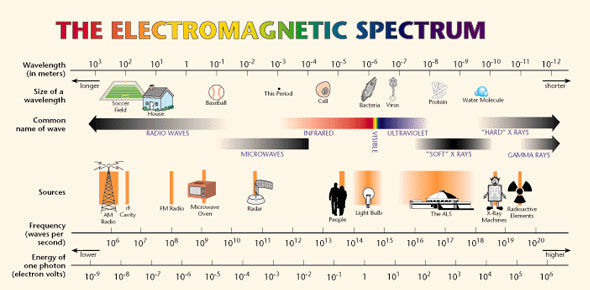# Em Spectrum - Gamma Rays

5 Questions | Total Attempts: 216SettingsThis quiz will test your basic understanding of Gamma Rays.

• 1.
Gamma rays have the lowest frequency in the EM spectrum.
• A.

True

• B.

False

• 2.
Gamma photons travel at the speed of sound.
• A.

True

• B.

False

• 3.
Can gamma rays be emitted by radioactive decay, nuclear fusion and nuclear fission?
• A.

Yes

• B.

No

• 4.
The three main types of radioactive decay are:
• A.

Alpha, beta, delta

• B.

Alpha, beta, gamma

• C.

Beta, gamma, delta

• 5.
Nuclear fussion is when an atom is split into two.
• A.

True

• B.

False

Related TopicsBack to top